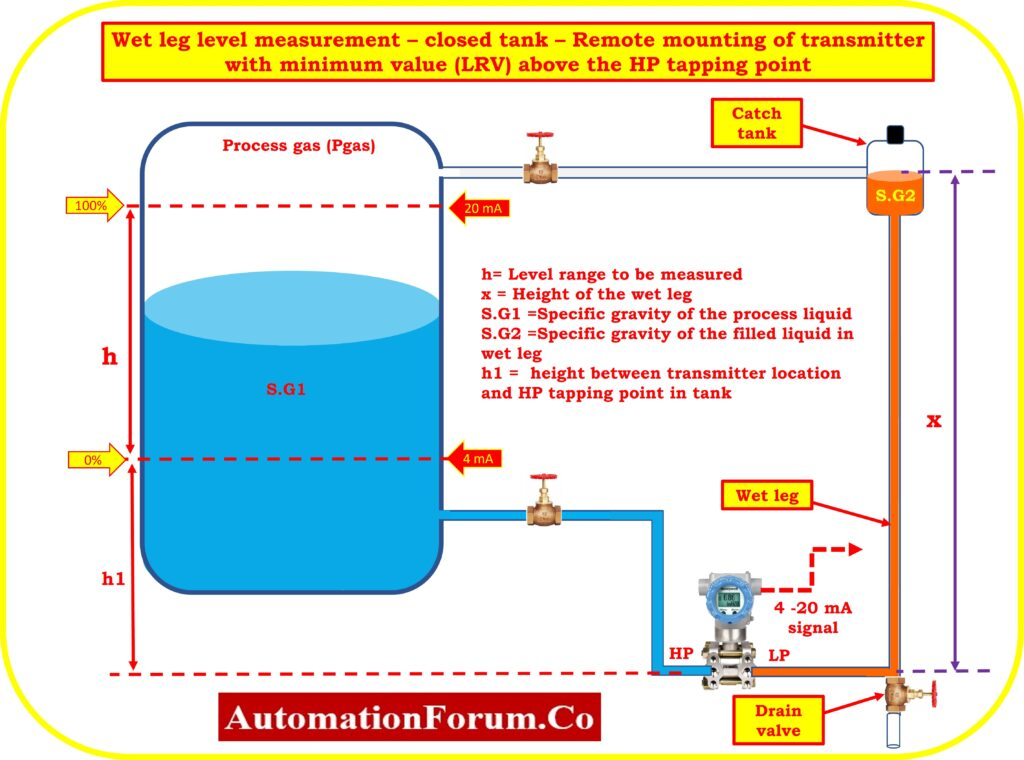# Closed tank level measurement – Wet Leg System – Transmitter installed in non-zero based remote mount applicationMounting the level transmitter exactly at the tank’s base level is not always practicable. In some instances, it may not be viable to install the level transmitter. Let’s say that in order to make it easier to maintain the level transmitter, it needs to be positioned Length (h1) below the bottom of the vessel.

At the beginning of the low pressure impulse line is a little catch tank. In the catch tank and wet leg, the gasoline vapors will condense. On the low pressure side of the level transmitter, a constant hydrostatic pressure is established due to the catch tank and the incline impulse connection line. Because of this pressure’s consistency, calibration makes it easy to take it into consideration.

h = Process Level range need to be measured

x = Height of the wet leg

h1= height between transmitter location and HP tapping point in tank

S.G1 = Specific gravity of the process liquid

S.G2 = Specific gravity of the filled liquid in wet leg

Pressure on H.P. side of transmitter P.HP = Pgas + (SG1*h)+(SG1*h1)

Pressure on LP side of the transmitter P.LP= Pgas + (SG2*x)

The following equation is used to compute the differential pressure of the transmitter:

DP = P.HP – P.LP

DP = {Pgas + (SG1*h) + (SG1*h1)} – {Pgas + (SG2*x)}

DP = (SG1*h) + (SG1*h1) – (SG2*x)

## LRV Calculation

Calculate the Differential pressure (DP) when the tank is empty (h=0). But Impulse line height (h1) between transmitter location and HP tapping point in tank filled with process liquig (SG1)

PH.P = {Pgas + (SG1*h) + (SG1*h1)}

PL.P = Pgas + (SG2*x)

LRV = PH.P. – PL.P

LRV = {Pgas + (SG1*h) + (SG1*h1)} – {Pgas + (SG2*x)}

LRV = {Pgas + (SG1*0) + (SG1*h1)} – {Pgas + (SG2*x)}

LRV = (SG1*h1) – (SG2*x)

## Estimating the URV

Determine the Differential pressure (DP) when the tank level has reached the maximum range with the process liquid (h)

PH.P = {Pgas + (SG1*h) + (SG1*h1)}

PL.P = Pgas + (SG2*x)

URV = PH.P. – PL.P

URV = {Pgas + (SG1*h) + (SG1*h1)} – {Pgas + (SG2*x)}

URV = {(SG1*h) + (SG1*h1)} – (SG2*x)

Range of the transmitter = {(SG1*h1) – (SG2*x)} to [{(SG1*h) + (SG1*h1)} – (SG2*x)]